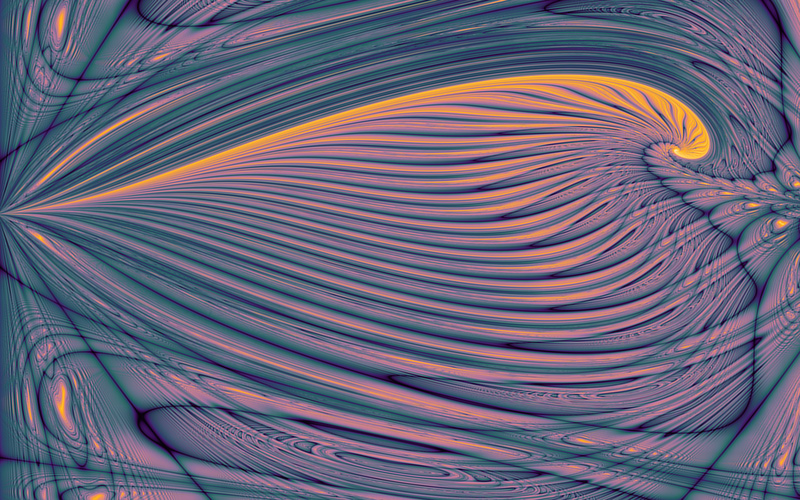Thorn Fractal

Written by Paul Bourke

Fractal attributed to Andrew Wayne Graff, alternatively named the "Secant Sea"
Contribution by Dane Vandeputte

Original: May 2004, Updated: May 2012

Sample C source code -- Contribution by Adam Majewski: t.c and p.c

This fractal is created by iterating the functions (xn,yn) and shading each pixel (x0,y0) depending on whether or how fast the series escapes to infinity. The parameter (cx,cy) is gives (slightly) different fractal images.

 xn xn+1 =+ cx cos(yn)
 yn yn+1 =+ cy sin(xn)cx = 0.102, cy = -0.04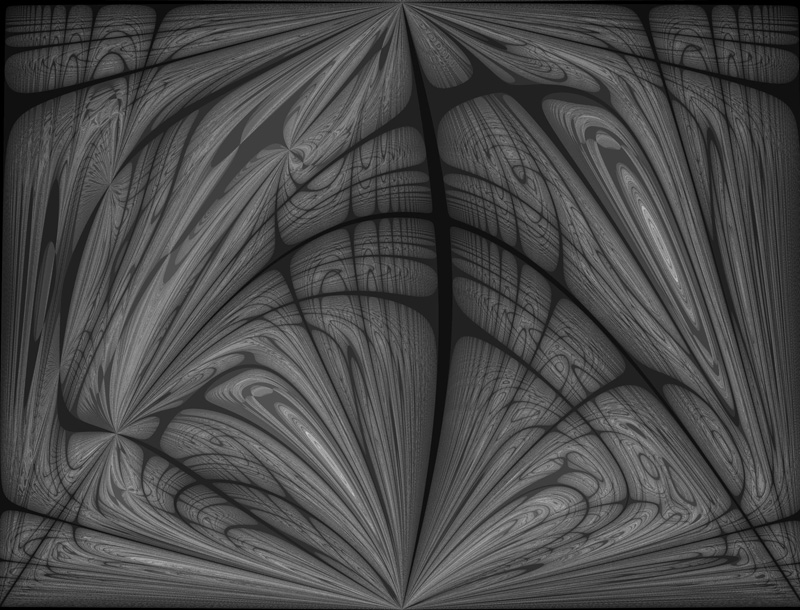cx = 1.098, cy = 1.402cx = 9.984, cy = 7.55cx = 0.662, cy = 1.086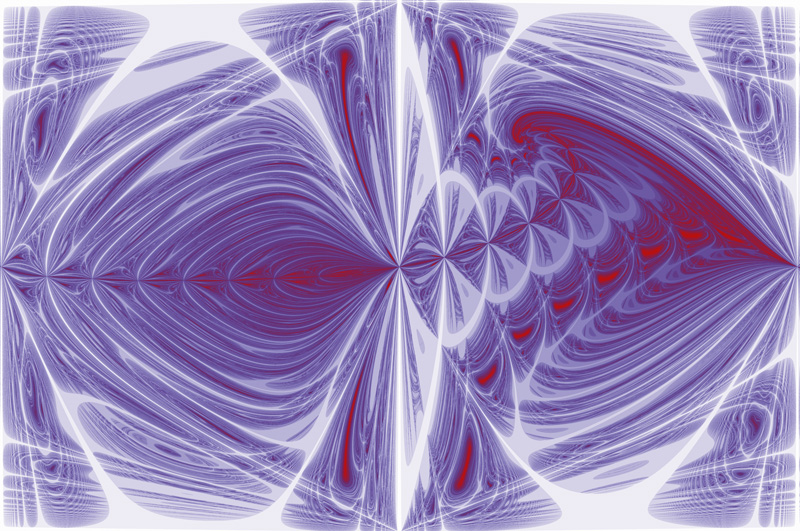cx = -0.354, cy = 0.162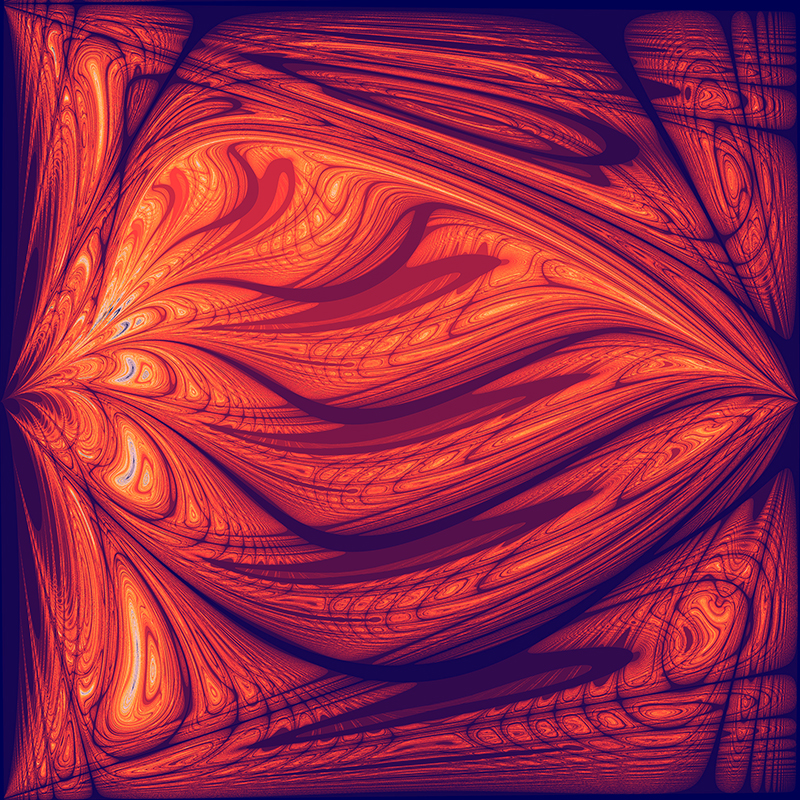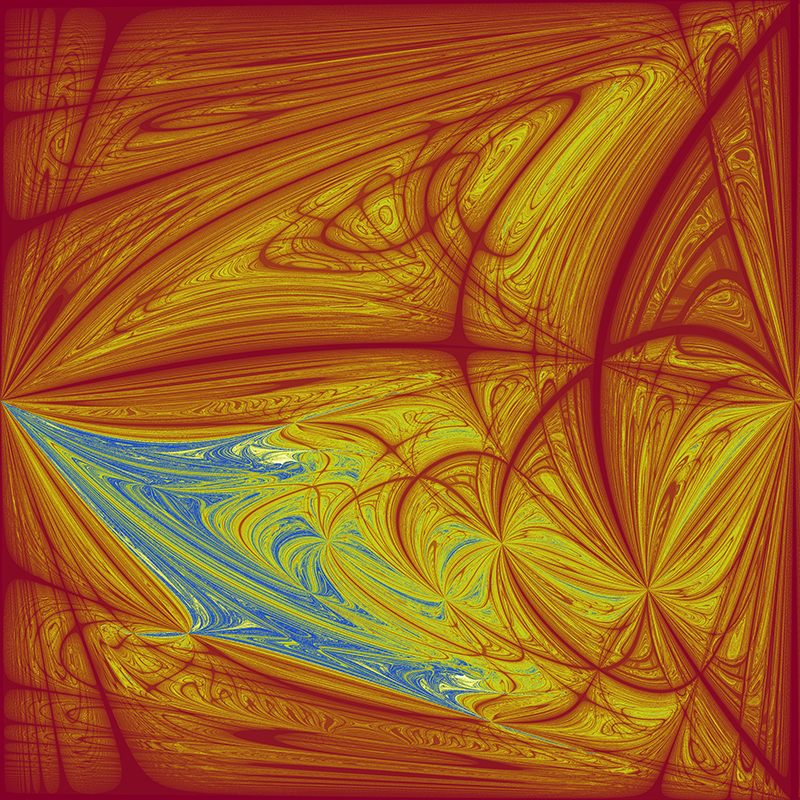Movie example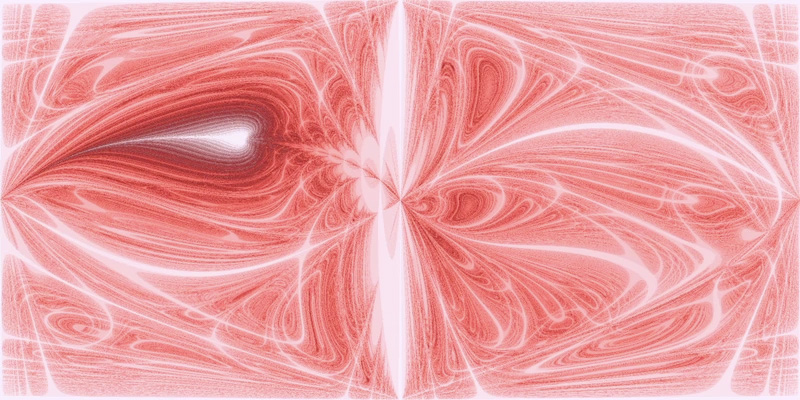cx and cy are moved around the unit circle, 1 degree steps.

The following images were rendered virtually at 131072x81920 pixels (~10.7 gigapixels) and down-sampled on-the-fly using a properly dilated Lanczos3 filter. Colouring was applied using a simple lookup table approach based on linearly interpolated iteration counts in the escape time algorithm (bailout radius: 10000, maximum iterations: 500, (cx, cy) = (0.1, -0.1)). CLAHE (Contrast Limited Adaptive Histogram Equalization) was then applied multiple times with varying block size, threshold, and amount to enhance local contrast.